nextnano.com  GUI: nextnanomat  Tool: nextnano++  Tool: nextnano³  Tool: nextnano.NEGF  Download | Search | Copyright | News | Publications  * password protected nextnano³ software2D Quantum corral

# nextnano3 - Tutorial

## Electron wave functions in a cylindrical well (2D Quantum Corral)

Author: Stefan Birner

`==> `This is the old website: A new version of this tutorial can be found here.

` -> 2DQuantumCorral_nn3.in / *_nnp.in  - `input file for the nextnano3 and nextnano++ software

## Electron wave functions in a cylindrical well (2D Quantum Corral)

A cylindrical InAs quantum well (diameter 80 nm) is surrounded by a cylindrical GaAs barrier (20 nm) which is surrounded by air.
The whole sample is 160 nm x 160 nm.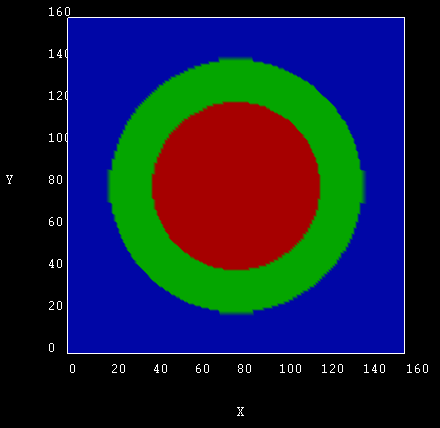We assume infinite GaAs barriers. This can be achieved by a circular quantum cluster with Dirichlet boundary conditions, i.e. the wave function is forced to be zero in the GaAs barrier. The electron mass of InAs is assumed to be isotropic and parabolic (me =  0.026 m0). Strain is not taken into account.

### Electron wave functions

The size of the quantum cluster is a circle of diameter 80 nm.

The following figures shows the square of the electron wave functions (i.e. psi²) of the corresponding eigenstates. They were calculated within the effective-mass approximation (single-band) on a rectangular finite-differences grid.

 1st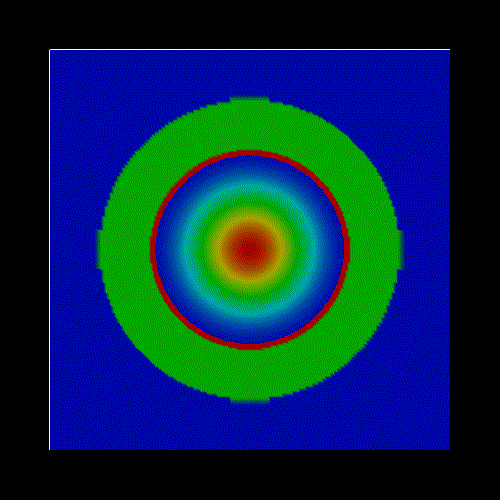2nd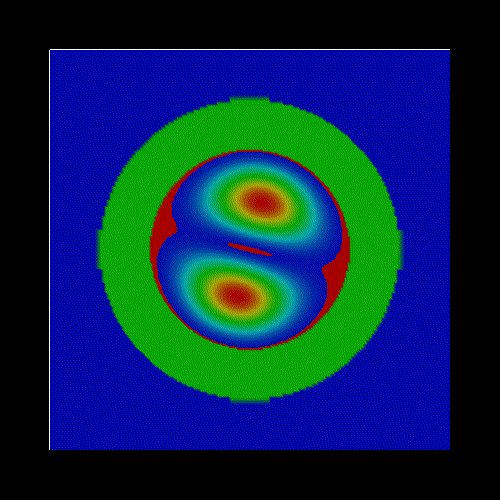3rd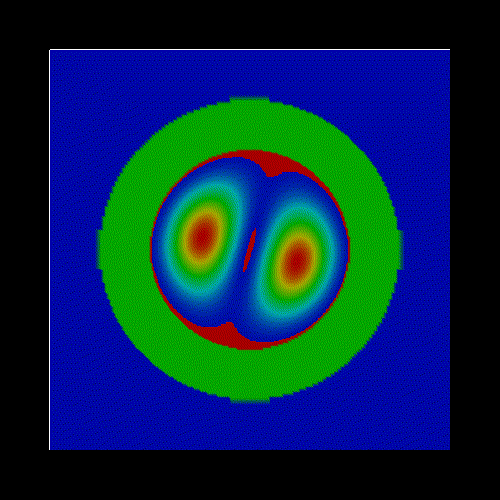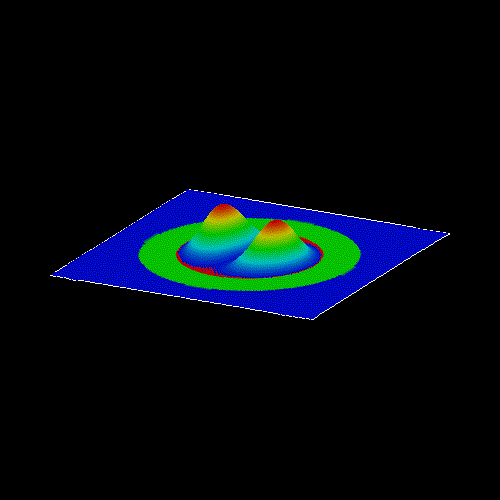4th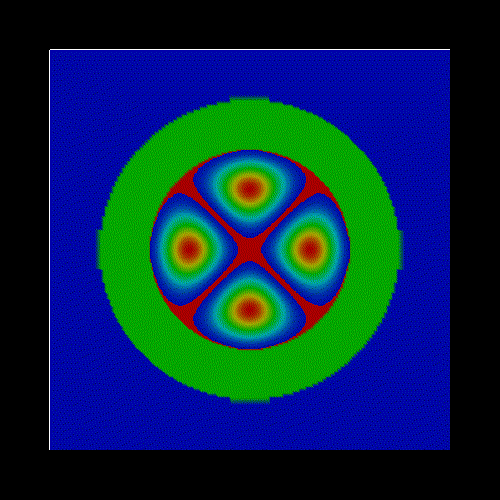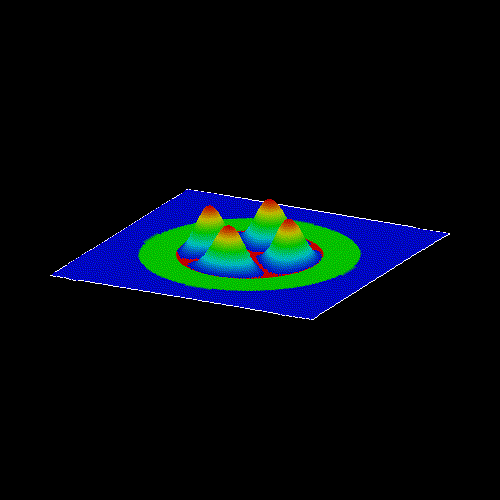5th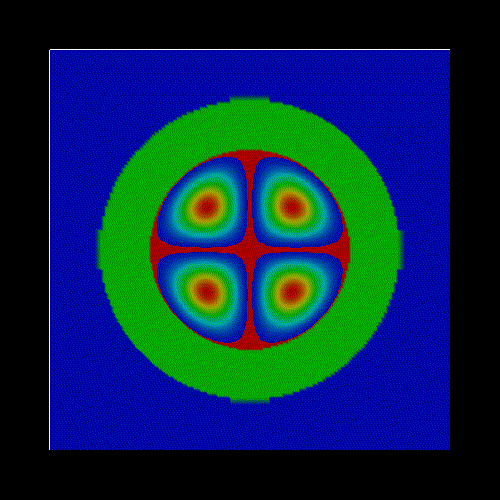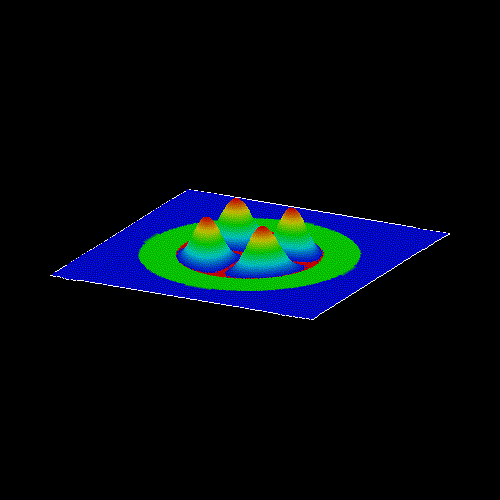6th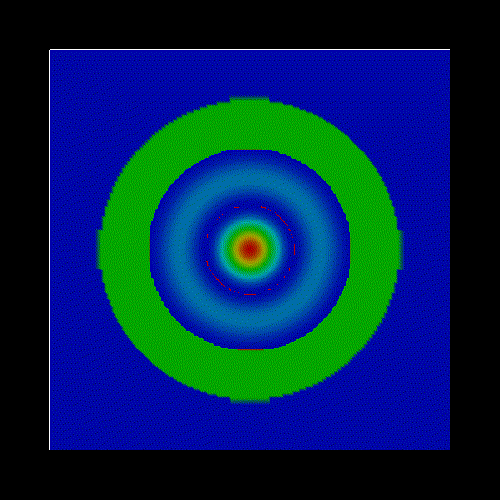15th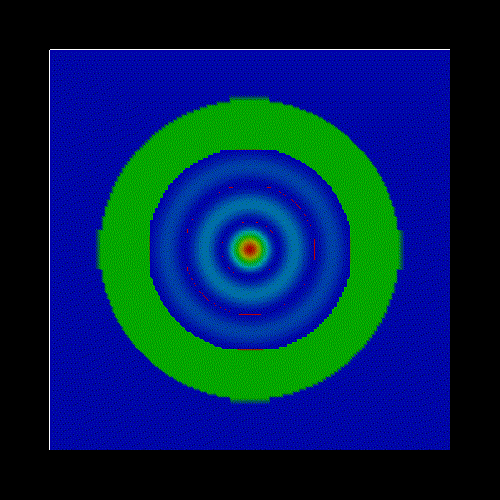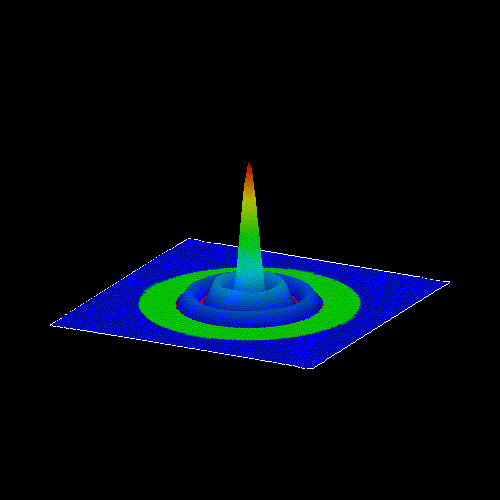20th22th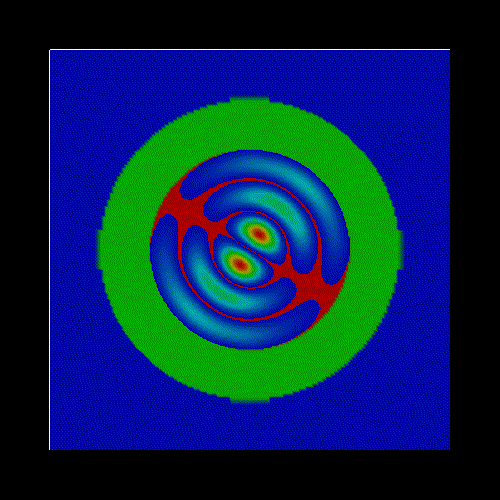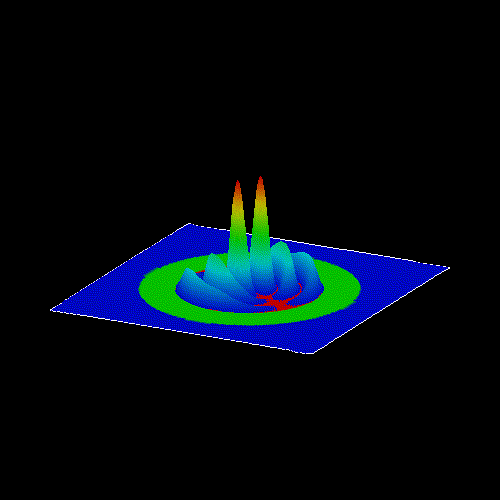The following figure shows the energy spectrum of the quantum corral. (The zero of energy corresponds to the InAs conduction band edge.)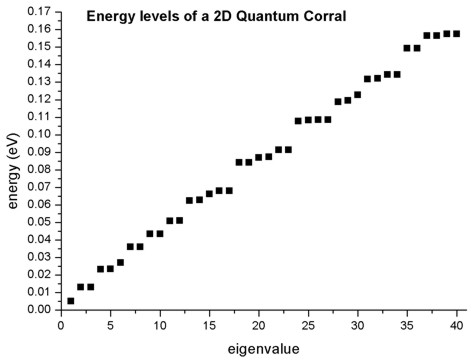One can clearly see the two-fold degeneracies of the states
2, 3
4, 5
7, 8
9, 10
11, 12
13, 14
16, 17
18, 19
20, 21
22, 23
24, 25
26, 27
28, 29
31, 32
33, 34
35, 36
37, 38
39, 40

The parameters of the quantum corral are the following:
- radius: a = 40 nm
- me =  0.026 m0
- V(r) = 0 for r < a
- V(r) = infinity for r > a

The energies are: Enl = hbar2 / (2me a2) * jl,n2

which can be simplified to this formula: Enl = 0.0381 / (me a2) eV * jl,n2
In the latter formula, me is dimensionless and a in units of [nm]. (In the formula above, me is in units of [m0] and a in [m].)

There is a formula to approximate jl,n:  jl,n = ( n + 1/2  | l |  - 1/4 ) pi
which is accurate as n` -> `infinity.
However, it seems that this formula is not accurate enough to compare the results to the nextnano³ results..

 Enl jl,n jl,n (approx.) [eV] (approx.) [eV] nextnano³ [eV] E1 = E10 2.405 = 0.765 pi 0.75 pi `0.005``08` `0.005``28` `0.005``11` E2 = E11 1.25 pi `0.01412` `0.01298` E3 = E1-1 1.25 pi `0.01412` `0.01298` E4 = E12 1.75 pi `0.02768` `0.02325` E5 = E1-2 1.75 pi `0.02768` `0.02325` E6 = E20 1.75 pi `0.02768` `0.02693` E7 = E21 2.25 pi `0.04576` `0.03597`

Further details about the analytical solution of the cylindrical quantum well with infinite barriers can be found in:

The Physics of Low-Dimensional Semiconductors - An Introduction
John H. Davies
Cambridge University Press (1998)

• Please help us to improve our tutorial. Send comments to``` support [at] nextnano.com```.